Soliton

A solution of a non-linear evolution equation which at every moment of time is localized in a bounded domain of space, such that the size of the domain remains bounded in time while the movement of the centre of the domain can be interpreted as the movement of a particle. The soliton solution of the Korteweg–de Vries equation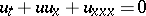given bydescribes such a solitary wave, and is uniquely determined by two parameters: the velocity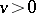and the position of the maximum at a fixed moment in time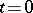,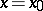. This equation also has-soliton solutions, which for large() can be written approximately as the sum ofterms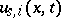, each of which is characterized by its velocity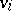and the position of its centre. For an-soliton solution, the set of velocities before collision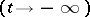and after collisionremains the same; there arise only shifts of the centres of soliton solutions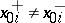. Many non-linear evolution equations in two independent variables have been found which possess solutions with the above properties. Thus, the soliton solution of the non-linear Schrödinger equation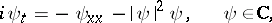is uniquely determined by four parameters, and that of the sine-Gordon equation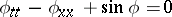by two parameters,: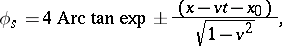and there is a double soliton (breather) which is defined by four parameters.

There is an analogous situation for the Boussinesq equation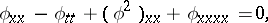the Hirota equation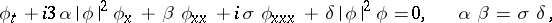and others. There are also physically interesting equations with a larger number of independent variables that have soliton solutions with the above properties. For example, a soliton of the Kadomtsev–Petviashvili equation (two space variables)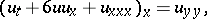localized inand, is equal to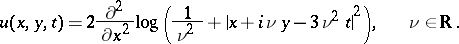In physics literature, the term "soliton" means a particle-like solution of a non-linear equation of classical field theory for which energy and momentum densities remain localized in a neighbourhood of some point of space at any moment in time. Sometimes, localization can occur near lines and surfaces. These localized solutions are also called kinks or monopoles. The search for solutions of this type involves topological considerations. In particular, for several models one can successfully construct a current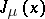whose divergence is equal to zero independently of the equations of motion, and the corresponding integral of motion (the topological charge)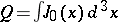gives a lower bound for the energy functional.